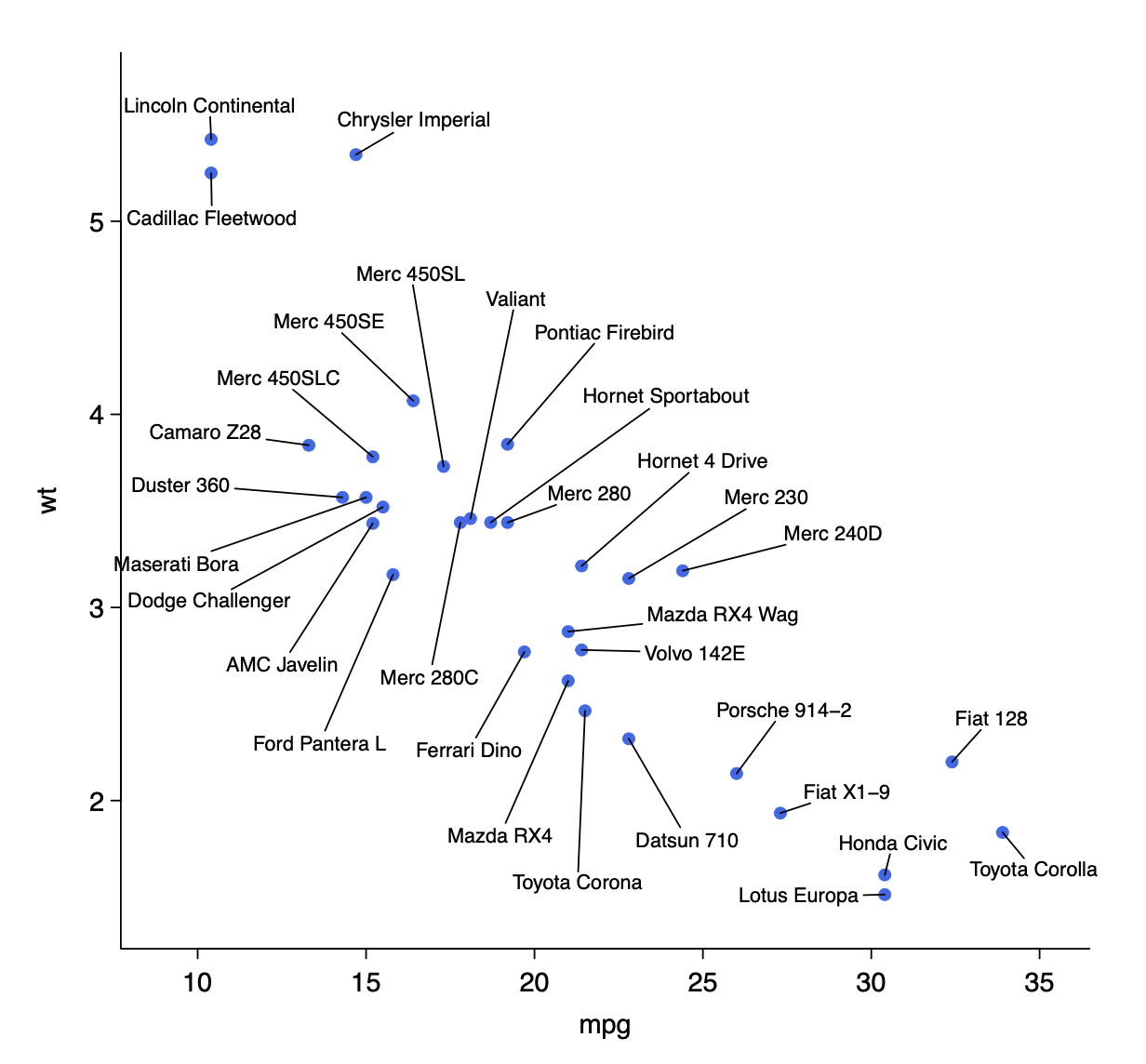# 1 Introduction

A common problem in R is labelling scatter plots with large numbers of points and/or labels. We provide a utility for easy labelling of scatter plots and quick plotting of volcano plots and MA plots for gene expression analyses. Using an interactive shiny and plotly interface, users can hover over points to see where specific points are located and click on points to easily label them. Labels can be toggled on/off simply by clicking. An input box and batch input window provides an easy way to label points by name. Labels can be dragged around the plot to place them optimally. Notably we provide an easy way to export directly to PDF for publication.

# 2 Installation

Install from CRAN

``````install.packages("easylabel")
library(easylabel)``````

Install from Github

``````devtools::install_github("myles-lewis/easylabel")
library(easylabel)``````

If you wish to use the optional `useQ` argument with `easyVolcano()` and `easyMAplot()`, you will need to install additional package `qvalue` from Bioconductor:

``````if (!requireNamespace("BiocManager", quietly = TRUE))
install.packages("BiocManager")
BiocManager::install("qvalue")``````

If you wish to use the optional `fullGeneNames` argument, you will need to install packages `AnnotationDbi` and `org.Hs.eg.db` from Bioconductor:

``````BiocManager::install("AnnotationDbi")
BiocManager::install("org.Hs.eg.db")``````

# 3 Scatter plots

Use `easylabel()` to open a shiny app and plot and label scatter plots. A table of the main data is supplied in the Table tab for easy viewing of data.

``````data(mtcars)
easylabel(mtcars, x = 'mpg', y = 'wt',
colScheme = 'royalblue')``````## 3.1 Export plot to PDF

• Hover over and click on/off genes which you want to label.
• When you have selected all your chosen genes, then drag gene names to move label positions.
• Click the save button to export a PDF in base graphics.

## 3.2 Export SVG from plotly

• Switch to SVG when finalised (only do this at last moment as otherwise editing can very slow, particularly with large numbers of points).
• Press the camera button in the plotly modebar to save image as SVG.

## 3.3 Export as plotly object

The `output_shiny` option enables users to switch between invoking the shiny app or directly outputting a plotly object. For example, to extract a plotly figure with draggable annotations:

``````data(mtcars)

p1 <- easylabel(mtcars, x = 'mpg', y = 'wt', col = 'cyl',
startLabels = rownames(mtcars)[mtcars\$gear == 5],
output_shiny = FALSE) %>%
layout(yaxis = list(zeroline = FALSE))

p2 <- easylabel(mtcars, x = 'mpg', y = 'drat', col = 'vs',
colScheme = c("dodgerblue", "orange"),
startLabels = rownames(mtcars)[mtcars\$gear == 5],
output_shiny = FALSE) %>%
layout(xaxis = list(zeroline = FALSE))

plotly::subplot(p1, p2, nrows = 2, shareY = TRUE, titleX = TRUE, margin = 0.05)``````